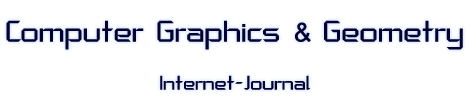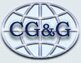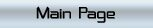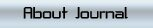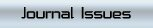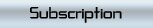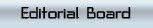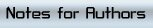### COMPUTER GRAPHICS & GEOMETRY

Issue Year: 2000
Date: Winter
Volume: 2
Number: 4
Pages: 25-49

 Article Name: ONE METHOD TO REDUCE COMPLEXITY OF MATRIX RADIOSITY ALGORITHM Authors: Victor A. Debelov, Igor M. Sevastyanov Address: Victor A. Debelov, Igor M. Sevastyanov Institute of Computational Mathematics and Mathematical Geophysics SB RAS, Russia Abstract: The given report is devoted to an original approach for reducing computational cost (memory and time) of the matrix radiosity algorithm. Such investigations are still quite actual because the algorithm requires significant amounts of memory and time. From other side it began to be applied in practice due to increasing power and memory of modern desktop computers. Recent efforts of computer graphics specialists were done in the following main directions. Adaptive scene subdivision (hierarchical radiosity methods) was elaborated in order to prevent the growth of the number of finite elements in acceptable limits. Grouping of scene objects cluster methods, when the light energy exchange is estimated between groups of scene elements (e.g., polygons) but between individual elements. All those approaches try to process the whole scene geometry in that or another way, and thus they do not simplify the original problem dramatically. Somewhat aside the method of imposters lies. It bases on geometric subdivision of an initial scene, but farther parts of a scene do not take part in calculations of light energy equilibrium, therefore it is lacking of photorealism. The given here method of geometric decomposition it is a quite new modification of the matrix radiosity algorithm. The scene is divided into parts (note, that it is done formally, i.e., without preliminary geometric analysis). Each part is processed independently on each other. Next step exchange of energy between parts. It is easy to see that an independence of parts allows: To solve more simple problems in each part. To process each part in parallel (coarse grain parallelism). To process each part by different modifications of radiosity algorithms. Possibility of recursive subdivision to gain a desirable rate of computational cost. Possibility to assemble a scene from precomputed parts. Note that in the given report we consider the just geometric decomposition of a scene in opposite to "decomposition" used in classical problems of computational mathematics. We show feasibility of the given approach for the case, when the scene is divided into parts by plane(s), really geometric bisection. The formulation and numerical experiments are presented too.

Issue contents